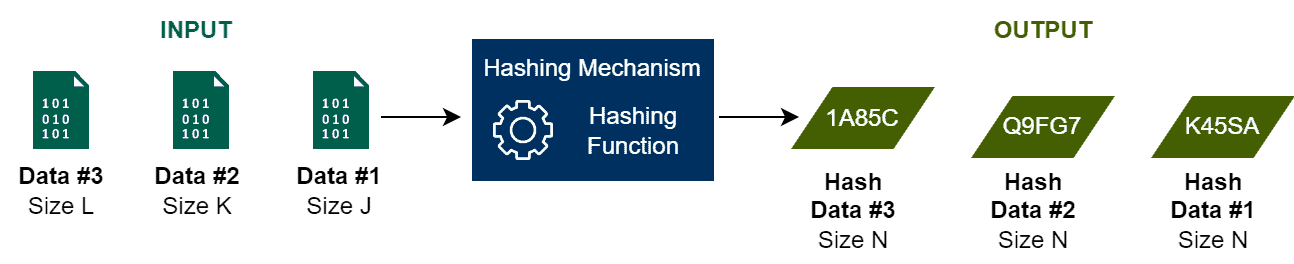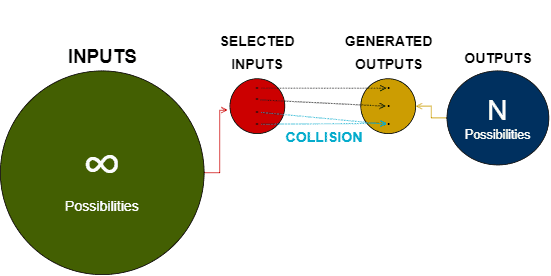## 1. Introduction

Hashing is the process of transforming a variable-length data input to a fixed-length output, called hash or digest. Hashing has different applications in modern computing, from storing passwords in databases to signing messages sent over the Internet.

However, as a consequence of how hashing works, collisions may occur. In summary, a collision is the generation of the same hash for different inputs. Hashing algorithms, in turn, can be designed to avoid collisions, being weakly or strongly collision-resistant.

In this tutorial, we’ll explore weak and strong hash collision resistance. First, we’ll have a brief review of hashes. So, we’ll particularly investigate hash collisions, detailing weak and strong collision resistance. Finally, we’ll present use cases where both weak and strong collision resistance are particularly relevant.

## 2. An Overview of Hashing

In summary, we can understand hashing as the process that maps any data to a fixed-length code. The overall process consists of providing raw data as input to a hashing mechanism, which processes it through a particular hashing function and generates the hash as output.

The following image depicts the hashing process at a high level:It is relevant to highlight that hashing functions are one-way processing. It means that these functions can compute hashes given the original data as input. However, they can not restore the original data given a hash code as input.

Hashing has several uses in computing. Among the most relevant hashing applications, we can cite password storing, data integrity verification, and the creation of key/value data structures:

• Password Storing: instead of keeping passwords in plain text, it is possible to store only a hash of the passwords in databases. So, if attackers get access to the database, they will need to process the hashes (typically, using brute force or rainbow tables strategies) to recover the passwords
• Data Integrity Verification: to enable data integrity verification, a know and open hashing mechanism generate a hash to a data provided as input. Thus, we can make this data available with its respective hash code. In this way, everyone who gets the data can recalculate the hash and compare it with the provided one: if they match, the data is correct
• Key/Value Data Structures: some programming languages provide data structures based on key/value relations. These data structures employ hash tables to keep generic data (values) linked to unique keys. So, we can access a specific stored data (value) requesting its respective key

Although the benefits, the intrinsic characteristics of hashing also raise some challenges. One of these challenges is the hash collision, discussed in the following section.

## 3. Hash Collisions

In short, a collision happens when different data inputs result in the same hash after being processed by a hashing mechanism. However, we should note here that collisions are not a problem but an intrinsic characteristic of the hashing mechanisms.

Collisions emerge due to the fact that hashing maps any input (regardless of its length) into a fixed-length code. So, since we have an infinite set of available inputs and a finite set o available outputs, the hashing mechanisms will eventually generate repeated hashes.

The image next depicts in high-level the described scenario:There exist techniques to solve collisions when they are not acceptable. For example, we can use replacement lists to shift the hash with a certain offset. This technique is typically used with hash tables.

Another technique consists of employing a hierarchy of hashing mechanisms in the system. Thus, if a collision occurs, we can recreate the hash with another hashing mechanism.

However, better than solving hashing collisions is avoiding them from happening. To accomplish that, the hashing mechanisms must guarantee particular properties regarding collision resistance.

In the following section, we will study the collision resistance properties.

## 4. Collision Resistance

Making hashing mechanisms resistant to collisions is a challenge primarily related to the design of the hashing function. Particularly, we should pay attention to the number of different hashes that a hashing function can generate. Furthermore, we should note the frequency that it generates each one of these hashes.

So, consider a specific problem that will require a hashing mechanism. A good hashing function to tackle the problem can generate enough different hashes to provide a unique hash to each input.

Moreover, given a set with any number of inputs, a well-designed hashing function returns a set of hashes where the most repeated hash has a minimum frequency difference with the least repeated one.

These characteristics are desirable to avoid collisions while using hashing mechanisms. But, we can explore the collision resistance in terms of avoiding collisions for specific inputs or arbitrary inputs. These features define weak and strong collision resistance.

### 4.1. Weak Collision Resistance

The definition of weak collision resistance is: given an input X and a hashing function H(), it is very difficult to find another input X’ on which H(X) = H(X’).

In other words, with an input X as the parameter, replicating the hash H(X) with another input X’ is not a trivial task.

### 4.2. Strong Collision Resistance

The main idea behind strong collision resistance is: given a hashing function H() and two arbitrary inputs X and Y, there exists an absolute minimum chance of H(X) being equal to H(Y).

In the case of strong collision resistance, we do not have a parameter to search for a collision as in the weak collision resistance.

In the strong collision resistance, we consider arbitrary inputs, and the chance of having a collision in this scenario is very low.

## 5. Use Cases of Hash Collision Resistance

There are many scenarios in which hash collision resistance becomes particularly relevant. In some of these scenarios, weak resistance is enough. However, other hashing scenarios may require strong collision resistance.

An example where weak collision resistance is typically enough is storing passwords as hashes in a database. In this case, only those who created the password know the input that generated the hash. So, the hashing mechanism protects the password due to the infeasibility of finding another input that generates the same hash.

On the other hand, an example where we need strong collision resistance is checking data integrity. In such a case, we have access to the original input that generated the hash. So, we can recalculate the hash and compare it with the provided one. For example, this process is usually employed when we digitally sign documents.

Thus, we believe in the improbability of finding an arbitrary input that generates the same hash. In such a way, we can trust that our data is a true copy of the original data.

## 6. Conclusion

In this tutorial, we studied hash collision resistance. At first, we had a brief review about hashes in general. Thus, we learned the central concepts about hash collisions. So, we explored the concepts regarding hash collisions resistance: weak and strong. Finally, we saw particular use cases of hash collision resistance in the real world.

We can conclude that hashing is an essential process for nowadays computing. However, regardless of the employed hashing mechanism/functions, collisions may occur. So, we must correctly design these mechanisms to avoid collisions, satisfying the weak or strong resistance properties.